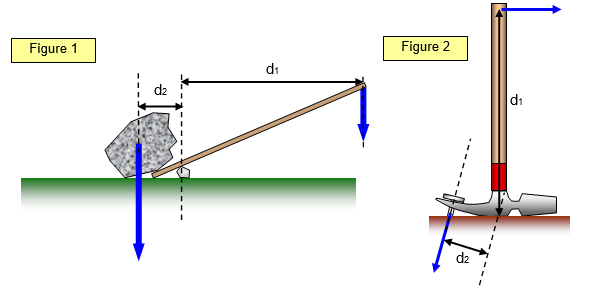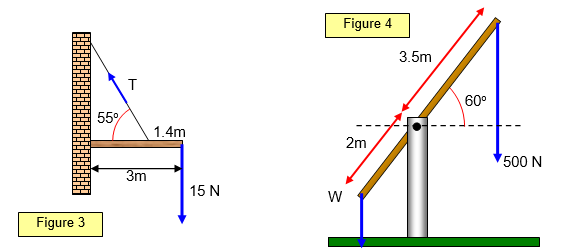# Moments 3

The following diagrams show the application of the principle of moments to two practical cases – a crowbar lifting a rock and a claw hammer being used to remove a nail from a piece of wood.Consider the forces in the two diagrams above. Notice how the angles are important in both cases.Figure 3:
Clockwise moment about P = 3 x 15 = 45 Nm
Anticlockwise moment = 45 = Tcos 35 x 1.6
Therefore: T = 34.3 N

Figure 4:
Wx2cos60 = 500x3.5cos60
Therefore W = 875 N and this is independent of the angle!

A VERSION IN WORD IS AVAILABLE ON THE SCHOOLPHYSICS USB